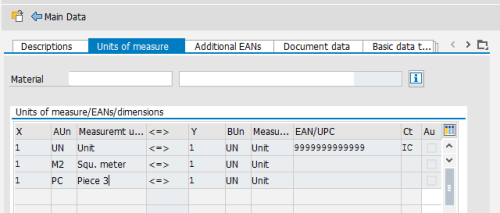# SAP Alternative Unit of Measure for Material

Before dealing with sap alternative unit of measure, let’s define what Base Unit of Measure for a SAP Material then we will explain the Alternative unit for Material.

Then I will explain how Alternative Units work for Material.

The system converts all the quantities you enter in other units of measure (alternative units of measure) to the base unit of measure.

## SAP Units of Measurement Overview

Unit of measure in which stocks of the material are managed.

You define the base unit of measure and also alternative units of measure and their conversion factors in the material master record.

It is usually refer in SAP with MEINS.

### SAP Units of Measurement Tables

All the Unit are stored in Standard Table T006.
In This Table T006, Details of the Unit and the kind of the unit are defined such as Nb of Decimal, Dimension key, Temperature, Pressure …

Check the table T006A (Assign Internal to Language-Dependent Unit) For Unit Description.

T006A-MSEHI is the Unit of Measurement and T006A-MSEHT is for Unit of Measurement Text.

### SAP Units of Measurement Conversion Function

IF you check the data element of MEINS (Unit of Measurement), it has in standard a conversion routine.

The conversion routine for MEINS are:

• CONVERSION_EXIT_CUNIT_INPUT Conversion exit for commercial (3-char) measurement unit INPUT
• CONVERSION_EXIT_CUNIT_OUTPUT Conversion exit for commercial (3-char) measurement unit OUTPUT

Actually, Unit of Measurement are defined in intern format and external format. This is can be helpful when looking for SAP Material Unit Conversion.

For example, the internal format for Box unit, is BOX and the external unit will be KAR (this is the commercial value).

This is can be found easily for Sap Material Unit Conversion function module with
CONVERSION_EXIT_CUNIT_INPUT or OUTPUT.

### SAP Units of Measurement on Material

The Most important Unit of Measurement for a Material is the Base Unit of Measure. It is the default Unit for Sales, Delivery and Stock when dealing with the Material.

The Value of SAP Base Unit for Measure for a Material can be found at MARA-MEINS.

## SAP Alternative Unit of Measure

A unit of measure that is defined in the SAP system in addition to the base unit of measure (BUn, base UoM).

For example, the base unit of measure might be “pallet” while the alternative unit might be “carton”.

### SAP Material : Alternative Units of Measure

In order to maintain or display this list, open the Material General Data (MM02 for Change or MM03 for Display), Go to Additional Data (on the top bar), then select the second tap Unit of Measure.

###Material Alternative Units of Measure Overview

Let’s try to explain how the Units of Measure works.

Unit of measure in which quantities can be entered alternatively to the base unit of measure / stock keeping unit.

To convert a quantity, whose unit of measure is not the same as the base unit of measure, into the base unit of measure, the system requires a quotient:

Quantity (in alternative unit of measure) = quotient * quantity (in base unit of measure)

In Order to define the Quotient = Numerator / Denominator

#### Denominator for conversion to base units of measure

Denominator of the quotient that specifies the ratio of the alternative unit of measure to the base unit of measure.

#### Numerator for Conversion to Base Units of Measure (Y)

Numerator of the quotient that specifies the ratio of the alternative unit of measure to the base unit of measure.

### SAP Material Alternative Units of Measure Table

The table where the alternative Units of measure, Numerator and Denominator is MARM (Units of Measure for Material).

• MEINH: Alternative Unit of Measure for Stock keeping Unit
• UMREZ: Numerator for Conversion to Base Units of Measure
• UMREN: Denominator for conversion to base units of measure

You can define an International Article Number (EAN/UPC) by alternative unit.

It is useless to look for the base unit in this table. Actually the Base Unit of measure is only on MARA-MEINS.

### Sap Alternative Unit : Convert Mater Quantities to Different Unit of Measure

In order to convert SAP Material Quantities from an unit of Measure to an other or an alternative unit, use the standard function module MATERIAL_UNIT_CONVERSION.

A sample use for MATERIAL_UNIT_CONVERSION is